# Train RL Agent for Lane Keeping Assist with Constraint Enforcement

This example shows how to train an reinforcement learning (RL) agent for lane keeping assist (LKA) with constraints enforced using the Constraint Enforcement block.

### Overview

In this example, the goal is to keep an ego car traveling along the centerline of a lane by adjusting the front steering angle. This example uses the same vehicle model and parameters as the Train DQN Agent for Lane Keeping Assist Using Parallel Computing (Reinforcement Learning Toolbox) example.

Set the random seed and configure model parameters.

```% Set random seed. rng(0); % Paramters m = 1575; % Total vehicle mass (kg) Iz = 2875; % Yaw moment of inertia (mNs^2) lf = 1.2; % Longitudinal distance from center of gravity to front tires (m) lr = 1.6; % Longitudinal distance from center of gravity to rear tires (m) Cf = 19000; % Cornering stiffness of front tires (N/rad) Cr = 33000; % Cornering stiffness of rear tires (N/rad) Vx = 15; % Longitudinal velocity (m/s) Ts = 0.1; % Sample time (s) T = 15; % Duration (s) rho = 0.001; % Road curvature (1/m) e1_initial = 0.2; % Initial lateral deviation from center line (m) e2_initial = -0.1; % Initial yaw angle error (rad) steerLimit = 0.2618;% Maximum steering angle for driver comfort (rad)```

### Create Environment and Agent for Collecting Data

In this example, the constraint function enforced by the Constraint Enforcement block is unknown. To learn the function, you must first collect training data from the environment.

To do so, first create an RL environment using the `rlLearnConstraintLKA` model. This model applies random external actions through an RL Agent block to the environment.

```mdl = 'rlLearnConstraintLKA'; open_system(mdl)```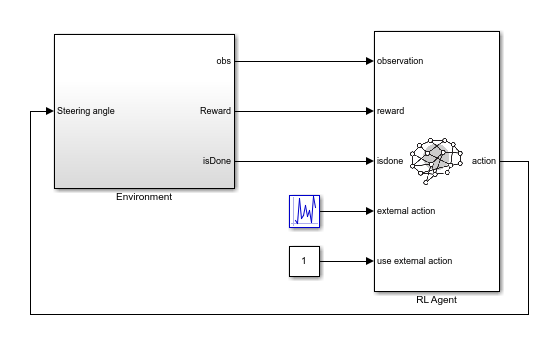The observations from the environment are the lateral deviation ${\mathit{e}}_{1}$, the relative yaw angle ${\mathit{e}}_{2}$, their derivatives, and their integrals. Create a continuous observation space for these six signals.

`obsInfo = rlNumericSpec([6 1]);`

The action from the RL Agent block is the front steering angle, which can take one of 31 possible values from -15 to 15 degrees. Create a discrete action space for this signal.

`actInfo = rlFiniteSetSpec((-15:15)*pi/180);`

Create an RL environment for this model.

```agentblk = [mdl '/RL Agent']; env = rlSimulinkEnv(mdl,agentblk,obsInfo,actInfo);```

Specify a reset function, which randomly initializes the lateral deviation and relative yaw angle at the start of each training episode or simulation.

`env.ResetFcn = @(in)localResetFcn(in);`

Next, create a DQN reinforcement learning agent, which supports discrete actions and continuous observations, using the `createDQNAgentLKA` helper function. This function creates a critic representation based on the action and observation specifications and uses the representation to create a DQN agent.

`agent = createDQNAgentLKA(Ts,obsInfo,actInfo);`

In the `rlLearnConstraintLKA` model, the RL Agent block does not generate actions. Instead, it is configured to pass a random external action to the environment. The purpose for using a data-collection model with an inactive RL Agent block is to ensure that the environment model, action and observation signal configurations, and model reset function used during data collection match those used during subsequent agent training.

### Learn Constraint Function

In this example, the safety signal is ${\mathit{e}}_{1}$. The constraint for this signal is ${-1\le \text{\hspace{0.17em}}\mathit{e}}_{1}\le 1$; that is, the distance from the centerline of the lane must be less than 1. The constraint depends on the states in $\mathit{x}$: the lateral deviation and its derivative, and the yaw angle error and its derivative. The action $\mathit{u}$ is the front steering angle. The relatinship between the states and the lateral deviation is described by the following equation.

`${\mathit{e}}_{1}\left(\mathit{k}+1\right)=\mathit{f}\left({\mathit{x}}_{\mathit{k}}\right)+\mathit{g}\left({\mathit{x}}_{\mathit{k}}\right){\mathit{u}}_{\mathit{k}}$`

To allow for some slack, set the maximum lateral distance to be 0.9.

The Constraint Enforcement block accepts constraints of the form ${\mathit{f}}_{\mathit{x}}+{\mathit{g}}_{\mathit{x}}\mathit{u}\le \mathit{c}$ . For the previous equation and constraints, the coefficients of the constraint function are:

`${\mathit{f}}_{\mathit{x}}=\left[\begin{array}{c}\mathit{f}\left({\mathit{x}}_{\mathit{k}}\right)\\ -\mathit{f}\left({\mathit{x}}_{\mathit{k}}\right)\end{array}\right],{\text{\hspace{0.17em}}\mathit{g}}_{\mathit{x}}=\left[\begin{array}{c}\mathit{g}\left({\mathit{x}}_{\mathit{k}}\right)\\ -\mathit{g}\left({\mathit{x}}_{\mathit{k}}\right)\end{array}\right],\text{\hspace{0.17em}}\mathit{c}=\left[\begin{array}{c}0.9\\ 0.9\end{array}\right]$`

To learn the unknown functions ${\mathit{f}}_{\mathit{x}}$ and ${\mathit{g}}_{\mathit{x}}$, the RL agent passes a random external action to the environment that is uniformly distributed in the range [–0.2618, 0.2618].

To collect data, use the `collectDataLKA` helper function. This function simulates the environment and agent and collects the resulting input and output data. The resulting training data has eight columns, the first six of which are the observations for the RL agent.

• Integral of lateral deviation

• Lateral deviation

• Integral of yaw angle error

• Yaw angle error

• Derivative of lateral deviation

• Derivative of yaw angle error

• Steering angle

• Lateral deviation in the next time step

For this example, load precollected training data. To collect the data yourself, set `collectData` to `true`.

```collectData = false; if collectData count = 1050; data = collectDataLKA(env,agent,count); else load trainingDataLKA data end```

For this example, the dynamics of the ego car are linear. Therefore, you can find a least-squares solution for the lateral-deviation constraints.

You can apply linear approximations to learn the unknown functions ${\mathit{f}}_{\mathit{x}}$ and ${\mathit{g}}_{\mathit{x}}$.

```% Extract state and input data. inputData = data(1:1000,[2,5,4,6,7]); % Extract data for the lateral deviation in the next time step. outputData = data(1:1000,8); % Compute the relation from the state and input to the lateral deviation. relation = inputData\outputData; % Extract the components of the constraint function coefficients. Rf = relation(1:4)'; Rg = relation(5);```

Validate the learned constraints using the `validateConstraintLKA` helper function. This function processes the input training data using the learned constraints. It then compares the network output with the training output and computes the root mean squared error (RMSE).

`validateConstraintLKA(data,Rf,Rg);`
```Test Data RMSE = 8.569169e-04 ```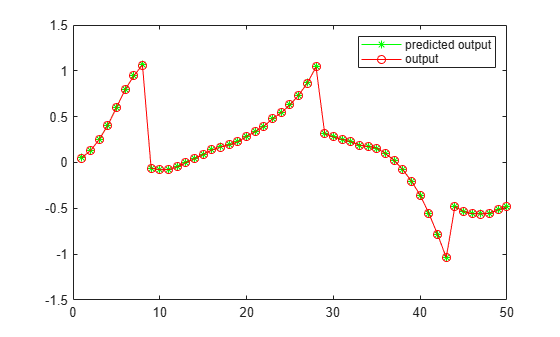The small RMSE value indicates successful constraint learning.

### Train RL Agent with Constraint Enforcement

To train the agent with constraint enforcement, use the `rlLKAWithConstraint` model. This model constrains the actions from the agent before applying them to the environment.

```mdl = 'rlLKAwithConstraint'; open_system(mdl)```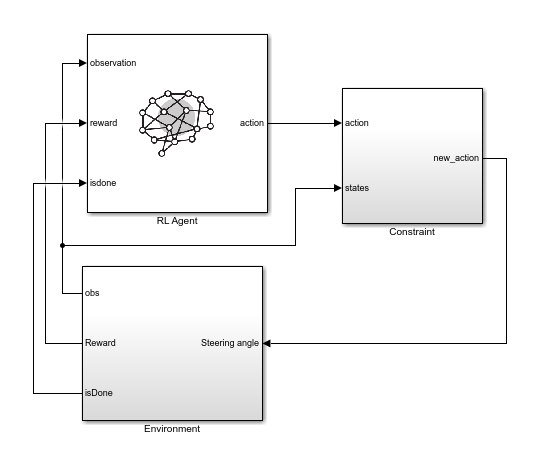To view the constraint implementation, open the Constraint subsystem. Here, the model generates the values of ${\mathit{f}}_{\mathit{i}}$ and ${\mathit{g}}_{\mathit{i}}$ from the linear constraint relations. The model sends these values along with the constraint bounds to the Constraint Enforcement block.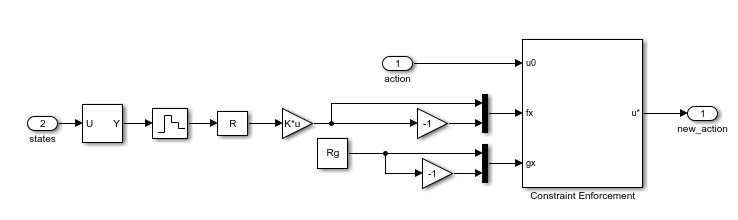Create an RL environment using this model. The observation and action specifications are the same as for the constraint-learning environment.

The Environment subsystem creates an `isDone` signal that is `true` when the lateral deviation exceeds a specified constraint. The RL Agent block uses this signal to terminate training episodes early.

```agentblk = [mdl '/RL Agent']; env = rlSimulinkEnv(mdl,agentblk,obsInfo,actInfo); env.ResetFcn = @(in)localResetFcn(in);```

Specify options for training the agent. Train the agent for at most 5000 episodes. Stop training if the episode reward exceeds –1.

```maxepisodes = 5000; maxsteps = ceil(T/Ts); trainingOpts = rlTrainingOptions(... 'MaxEpisodes',maxepisodes,... 'MaxStepsPerEpisode',maxsteps,... 'Verbose',false,... 'Plots','training-progress',... 'StopTrainingCriteria','EpisodeReward',... 'StopTrainingValue',-1);```

Train the agent. Training is a time-consuming process, so for this example, load a pretrained agent. To train the agent yourself instead, set `trainAgent` to `true`.

```trainAgent = false; if trainAgent trainingStats = train(agent,env,trainingOpts); else load rlAgentConstraintLKA agent end```

The following figure shows the training results.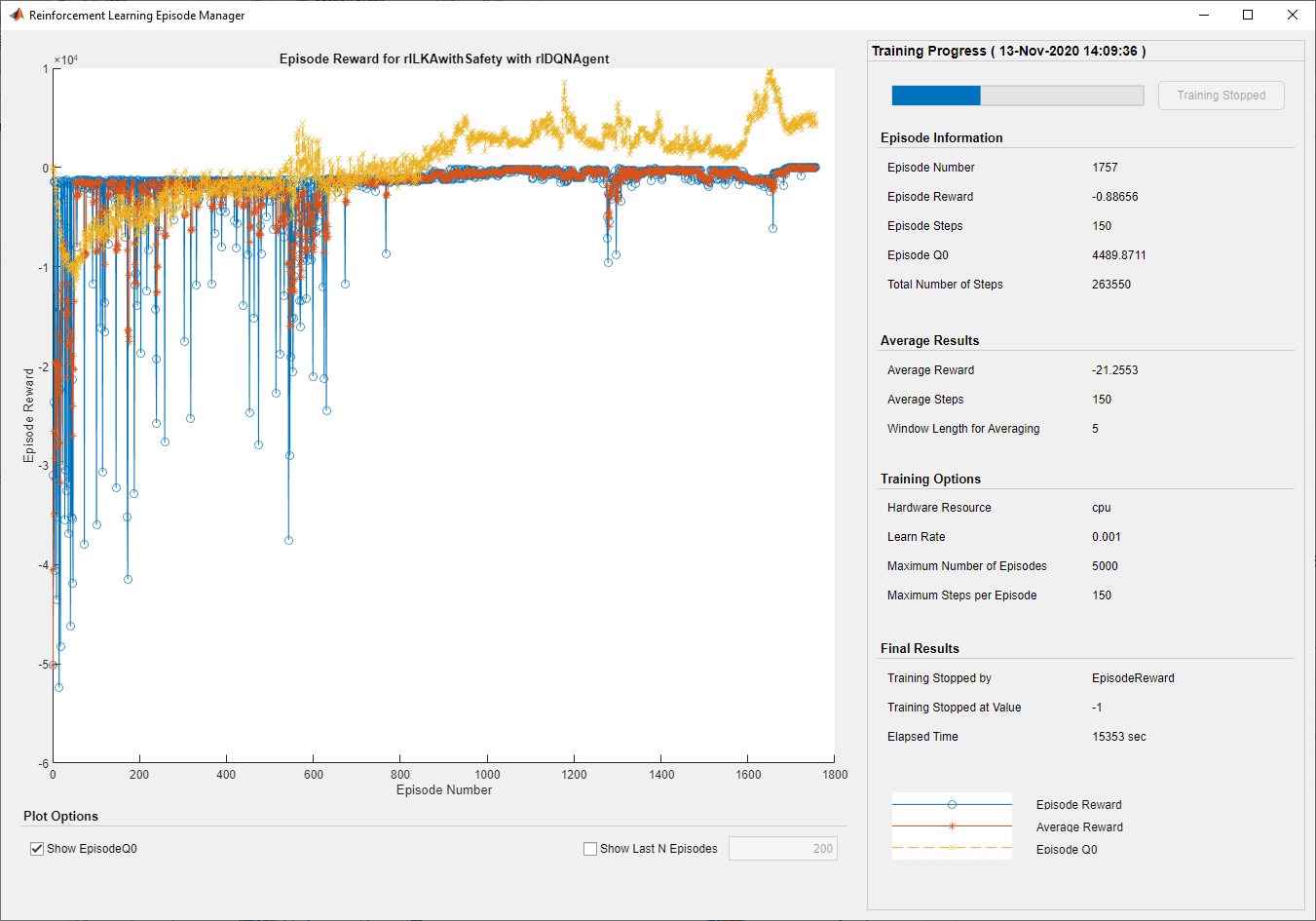Since Total Number of Steps equals the product of Episode Number and Episode Steps, each training episode runs to the end without early termination. Therefore, the Constraint Enforcement block ensures that the lateral deviation never violates its constraints.

Run the trained agent and view the simulation results.

```e1_initial = -0.4; e2_initial = 0.2; sim(mdl);```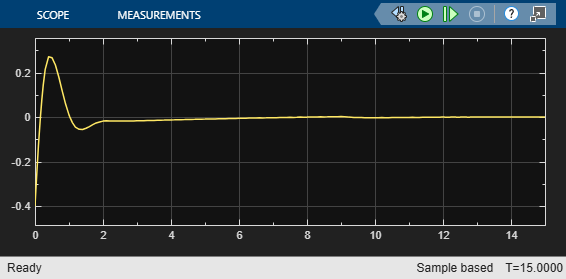```bdclose('rlLearnConstraintLKA') bdclose('rlLKAwithConstraint')```

### Local Reset Function

```function in = localResetFcn(in) % Set initial lateral deviation to random value. in = setVariable(in,'e1_initial', 0.5*(-1+2*rand)); % Set initial relative yaw angle to random value. in = setVariable(in,'e2_initial', 0.1*(-1+2*rand)); end ```

## Related Topics